The Plot 3D Toolbar

Figure 4-7. The QtiPlot Plot 3D ToolbarTable 4-27. 3D Plot toolbar commands.

IconCommandKeyDescriptionplot -> Frame Draw only the three axes.plot -> Box Draw the three axes and the 3D box around the plot.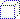plot -> No axes Doesn't draw the axes nor the box.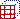plot -> Front Grid Draw a grid on the front panel.plot -> Back Grid Draw a grid on the back panel.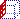plot -> Left Grid Draw a grid on the left panel.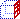plot -> Right Grid Draw a grid on the right panel.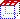plot -> Ceiling Grid Draw a grid on the top panel.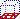plot -> Floor Grid Draw a grid on the bottom panel.plot -> Enable perspective Enables/Disables the 3D perspective mode.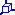plot -> Reset rotation Resets the rotation of the 3D plot to the default values.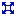plot -> Fit frame to window Finds the best layout of the 3D plot fitting the window size. It readjusts the length of the axis ticks to a default value.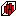plot -> Bars Style Changes the styles of the bars.plot -> Dots Draw the 3D scatter points with the dot style.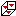plot -> Cones Draw the 3D scatter points with the cone style.plot -> Cross Hairs Draw the 3D scatter points with the cross-hairs style.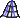plot -> 3D Wire Frame Draw a surface with the wireframe style.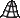plot -> 3D Hidden Lines Draw a surface with the mesh style (with hidden lines).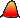plot -> 3D Polygons Draw a surface with the polygons style.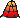plot -> 3D Wire Surface Draw a surface with the mesh+polygons style.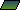plot -> Floor Data Projection Draw a projection of the plot on the floor.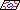plot -> Floor Isolines Draw an isolines projection on the floor.plot -> Empty Floor Draw an empty floor.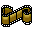plot -> Animation Enables/Disables animation.